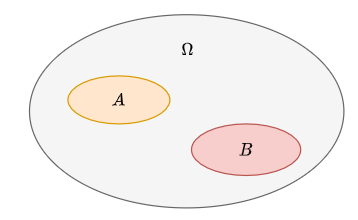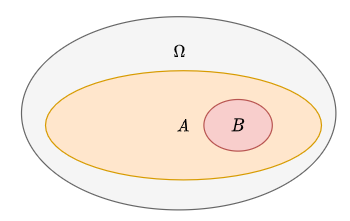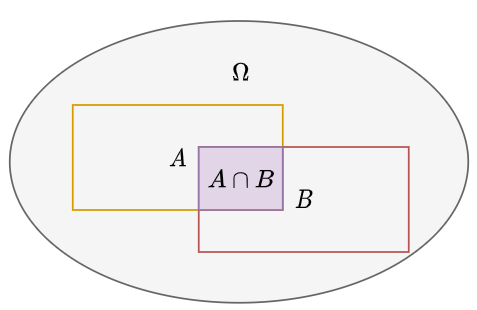Site Overlay

# 条件概率、全概率公式和贝叶斯定理

## 正文

### 条件概率

#### 条件概率的定义

$$P(X=a|Y=b) = \dfrac{P(X=a, Y=b)}{P(Y=b)}$$

#### 条件概率的几何意义### 概率空间

$F$ 是 $\Omega$ 的幂集的非空子集，称为事件集（注意，因为是幂集的子集，所以它的所有元素也都是集合）。满足以下性质：

1. 包含不可能事件（$\Phi \in F$）
2. $\Sigma \in F \to \Sigma^c \in F$，其中 $\Sigma \in F$，$c \in \mathbb{Z}^+$。
3. 可列可加（$\Sigma_1, \Sigma_2, \cdots, \Sigman \in F \to \cup{i=1}^n\Sigma_i \in F$）

$P$ 是概率测度。记作 $Pr$ 或 $\mathbb{P}$。满足以下性质：

1. $\forall \Sigma \in F, Pr(\Sigma)\in[0,1]$。
2. $Pr(\Omega) = 1$
3. 互斥事件 $\Sigma_1, \Sigma_2, \cdots, \Sigma_n \in F$ 的联合概率等于各自概率的乘积。

### 全概率公式

#### 全概率公式

$P(A) =\sum_n P(AB_n)$。

$P(A,B_n) = P(A|B_n) P(B_n)$

$P(A) = \sum_n P(A|B_n) P(B_n)$ 此为全概率公式

### 计算条件概率的替代方法——贝叶斯定理

1. $P(A|B) = P(B|A) P(A) / P(B)$
2. $P(B|A) = P(A|B) P(B) / P(A)$

#### 最大似然估计（MLE）

$$P(O|I_1) = \frac{P(O, I_1)}{P(I_1)}$$

$$P(O|I_1) = P(I_1 | O) P(O) / P(I_1)$$

### 贝叶斯定理的应用

#### 样本训练

word1, 0.02, 0.4
word2, 0.01, 0.06
...

#### 计算方法和实例

$$P(S|w_1) = \frac{P(w_1|S) P(w_1)}{P(S)}$$

$$P(w_1) = P(w_1|S)P(S) + P(w_1|H)P(H)$$

$$P(S|w_1) = \frac{P(w_1|S)}{P(S)}[P(w_1|S)P(S) + P(w_1|H)P(H)]$$

$$P(S|w_1) = (0.4/0.5)(0.4\times0.5+0.02\times0.5)=0.4\times 0.42=0.168$$

$$P(y \mid x_1, \dots, x_n) = \frac{P(y) P(x_1, \dots x_n \mid y)}{P(x_1, \dots, x_n)}$$

$$P(x_i | y, x1, \dots, x{i-1}, x_{i+1}, \dots, x_n) = P(x_i | y)$$

$$P(y \mid x_1, \dots, xn) = \frac{P(y) \prod{i=1}^{n} P(x_i \mid y)}{P(x_1, \dots, x_n)}$$

## 参考文献

[Bayes' theorem in Artificial intelligence](https://www.javatpoint.com/bayes-theorem-in-artifical-intelligence#:~:text=Bayes' theorem is also known,probabilities of two random events.)

## 1 thought on “条件概率、全概率公式和贝叶斯定理”

1.如何如何 says:

加油，好好学，概率论、统计、随机过程、高数、线性代数、优化是做ML的必修课。